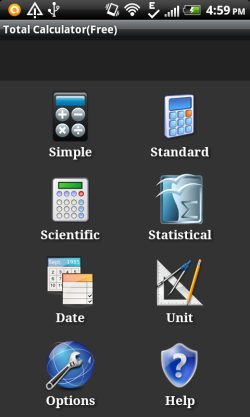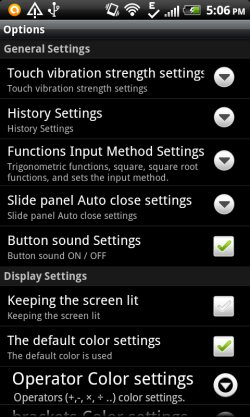Editor Ratings:
User Ratings:
[Total: 0 Average: 0]

Total Calculator is a free Android calculator app that provides multiple calculators on Android. This Android calculator app provides simple calculator, standard calculator, scientific calculator, statistical calculator, date  calculator, and Unit calculator (to convert between units).

I truly like the app, as you don’t need any other calculator apps for Android other than this calculator app for Android.The app works better on Android devices with big screen size. On launching the app, you will be asked to tap on the calculator type which you want to use.

The unique part of this Total Calculator app which I found is the date and units calculator. Till now, I didn’t find any calculator which allows me to do date and units calculation. Date calculator of the app allows you to calculate difference in days and months from any starting and end date. Just select the start date and the end date and leave rest on date calculator of the app. You can also calculate the exact date after adding days to a specified date. The app will show you the exact or total number of years, months, and days.

Unit calculator of the allows you to calculate units as per the length, area, velocity, and time. Enter the number and select a unit to convert the number in other units. You will see the list of different units converted.

## What I Like About Total Calculator App?

Total Calculator app defines its name in a perfect way as the app provides almost all types of calculators. After installing the app, you don’t need any other app to make calculations of any type.

The default calculator of your Android phone does not allows you to make advanced calculations like scientific calculations, date, units, and simple calculation. Also, the user interface of the calculator app is simple to use.

## Various Calculators Included in Total Calculator App for Android:

Simple Calculator:

Simple Calculator of the app provides basic calculator to make simple calculations which include division, multiplication, subtraction, addition, and calculation of percentage on Android. The calculator displays simple number pad to enter the number and make simple mathematical calculations.

Standard Calculator:

Standard Calculator works like an add-on to simple calculator. The additional mathematical functions include brackets, memory buttons, plus/minus button, square root, and percentage functions. You can easily use these functions while doing calculations on your Android phone.

Scientific Calculator:

Scientific Calculator provides complete calculator including scientific functions. Scientific calculator will be launched in landscape mode on Android. Along with the scientific functions, the app also displays functionality of simple and standard calculator on Android.

Statistical Calculator:

Statistical Calculator of the app provides statistical functions to make statistical calculation. Statistical calculator does not provide the functions of simple and standard calculator. You can only perform basic statistical calculations on your Android phone.

Date Calculator:

Date Calculator allows you to calculate difference between two dates. The difference between two dates are displayed in year, month, week, and day. You don’t have to take a notepad and calculate the difference in two dates. You just have to enter the start date and the end date and the app will automatically calculate the difference. You can even calculate the exact date by adding desired number of days to a particular date.

Unit Calculator:

Unit Calculator allows you to calculate a particular measurement in multiple units. The app allows you to calculate length, area, velocity, and time. The units are given accordingly in unit calculator app in different tabs on your Android phone.

## Features Of Total Calculator App:

• Provides multiple calculators which include simple, standard, scientific, and statistical calculator.
• The app even provides date and unit calculator.
• Simple to use interface.
• Touch vibration feature.• Button sounds.
• You can customize operator colors and bracket colors.
• Works good on big screen Android devices.
• You can customize the history settings as to keep your calculation records.

## Install Total Calculator App: